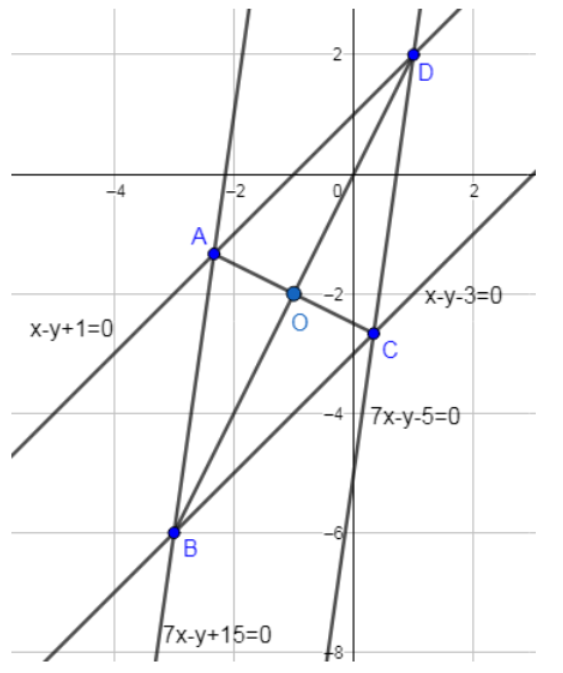Courses
Courses for Kids
Free study material
Free LIVE classes
MoreLIVE
Join Vedantu’s FREE Mastercalss

# Two sides of a rhombus are along the lines x-y+1 = 0 and 7x-y-5 = 0. If its diagonals intersect at (-1, -2), then which one of the following is a vertex of this rhombusa) (-3, -8)b) $\left( \dfrac{1}{3},\dfrac{-8}{3} \right)$ c) $\left( \dfrac{-10}{3},\dfrac{7}{3} \right)$ d) ( -3, -9)Verified
335.1k+ views
Hint: Find the perpendicular distance to both the lines and then double the distance and find parallel lines that make the other side. After that, find their intersection. Formula for finding the perpendicular distance is: d = $\dfrac{\left| am+bn+c \right|}{\sqrt{{{a}^{2}}+{{b}^{2}}}}$ and formula for finding distance between parallel lines is = $\dfrac{\left| {{c}_{1}}-{{c}_{2}} \right|}{\sqrt{{{a}^{2}}+{{b}^{2}}}}$.

We are given 2 lines in the question and the point where the diagonals intersect. Now we know that in a rhombus opposite sides are parallel and our equations are intersecting so they must belong to adjacent sides.
First, we find the perpendicular distance to both the lines from the given point.
The formula is: - d = $\dfrac{\left| am+bn+c \right|}{\sqrt{{{a}^{2}}+{{b}^{2}}}}$
Where d is the distance and a, b are coefficients of equation of line and (m,n) are the coordinates of the external point
Line 1: - x-y+1=0 and (-1, -2)
d = $\dfrac{\left( -1+2+1 \right)}{\sqrt{2}}$ = $\sqrt{2}$
So, equation of line parallel to this one and other side of the Rhombus is
Distance between parallel lines = $\dfrac{\left| {{c}_{1}}-{{c}_{2}} \right|}{\sqrt{{{a}^{2}}+{{b}^{2}}}}$
$2\sqrt{2}=\dfrac{\left| 1-{{c}_{2}} \right|}{\sqrt{2}}$
$4=\left| 1-{{c}_{2}} \right|$
$c_2$ = 5, -3.
To find out the point we have to check the distance by using both values of c and that gives us
$c_2$ = -3. Equation of parallel line => x-y-3 = 0
Similarly, we find the equation for other line which comes out to be
7x-y+15 = 0
Our final diagram after plotting all the equations and point looks like this:In the above figure the given lines are drawn and the formed rhombus is shown having vertices A, B, C, D and the point of intersection of the diagonals AC and BD is O which is at (-1,-2). The point D is at the intersection of the lines x-y+1=0 and 7x-y-5=0 and the point B is at the intersection of the lines x-y-3=0 and 7x-y+15=0.
Intersection of the line 7x-y-5 = 0 and x-y-3 = 0 is at the point C whose coordinates are given by $\left( \dfrac{1}{3},-\dfrac{8}{3} \right)$.

So, the correct answer is “Option B”.

Note: Solve carefully while opening the absolute value brackets as there is a high possibility of not checking both the cases which may lead to neglecting the actual right answer. You can also solve it by joining the diagonal with the given vertex and making the triangle and thus we know the length and angle and we know it’s a right triangle so we use trigonometry to find other vertices.
Last updated date: 25th Sep 2023
Total views: 335.1k
Views today: 3.35k